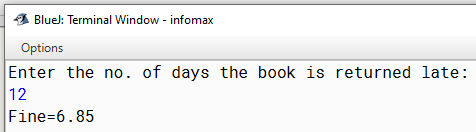# Java program to calculate the fine assuming that a book is returned N days late

A library charges a fine for books returned late. Following are the fines:

First five days : 40 paise per day.

Six to Ten days : 65 paise per day.

Above ten days : 80 paise per day.

Design a program to calculate the fine assuming that a book is returned N days late.

`Solution`

``````import java.util.*;
class Fine
{
public static void main(String arr[])
{
int N;
float f;
Scanner sc=new Scanner(System.in);
System.out.println("Enter the no. of days the book is returned late:");
N=sc.nextInt();
if (N<=5)
f=0.40f*N;
else if (N<=10)
f=0.40f*5+(N-5)*0.65f;
else
f=0.40f*5+0.65f*5+(N-10)*0.80f;
System.out.println("Fine="+f);
}
}``````
`Output`